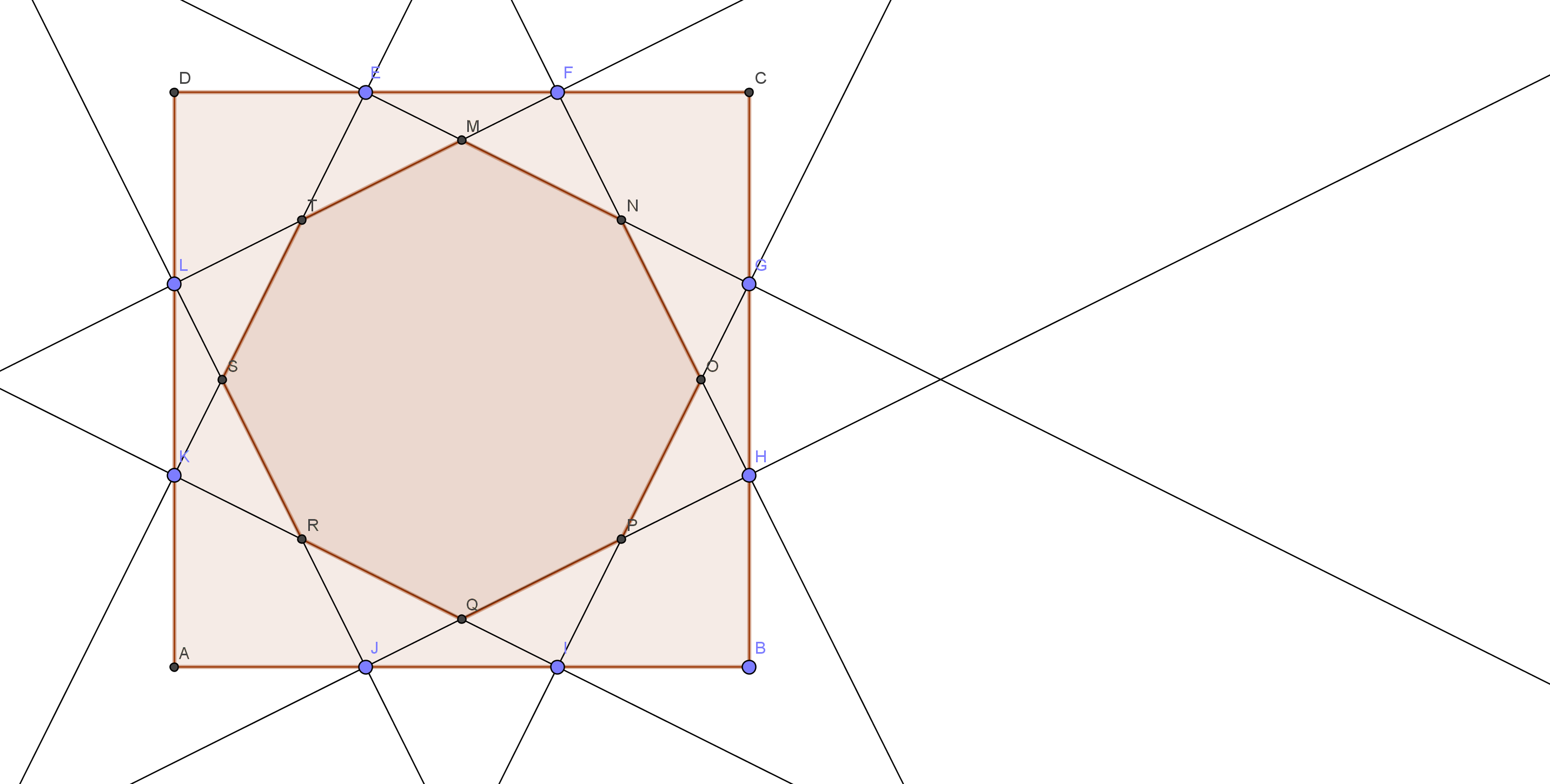# Midpoint? What About Thirds?

Geometry Level 4$ABCD$ is a square with a side length of 18. Divide each side​ into three equal parts, and mark the points $E,F,G,H,I,J,K,L$ in a clockwise manner starting from the vertex $D$. Then, connect the points to get a not-necessarily-regular octagon as shown in the figure above. What is the area of the octagon?

Bonus: You may notice that the ratio between the segments of all the sides are all $1:1:1$. Now, generalize this by considering the case of $1:k:1$, where $k\in\mathbb{N}$.

###### You may wish to try Part 2 and Part 3 of this trilogy.
×

Problem Loading...

Note Loading...

Set Loading...